# Area Of Shape - Definition with Examples

The Complete K-5 Math Learning Program Built for Your Child

• 40 Million Kids

Loved by kids and parent worldwide

• 50,000 Schools

Trusted by teachers across schools

• Comprehensive Curriculum

Aligned to Common Core

## Area of Shapes

Geometric shapes are the figures with a set of points connected by lines resulting in a closed figure.

For example, triangle, square, rectangle and quadrilateral are shapes with 3 and 4 points connected by lines. Shapes with bounded curves don’t have sides but have the circumference.Mirrors of different shapes with sides and curves.

## Area

The area of a shape is the “space enclosed within the perimeter or the boundary” of the given shape. We calculate the area for different shapes using math formulas.

In the following pictures, the shaded region denotes the area for the respective shapes.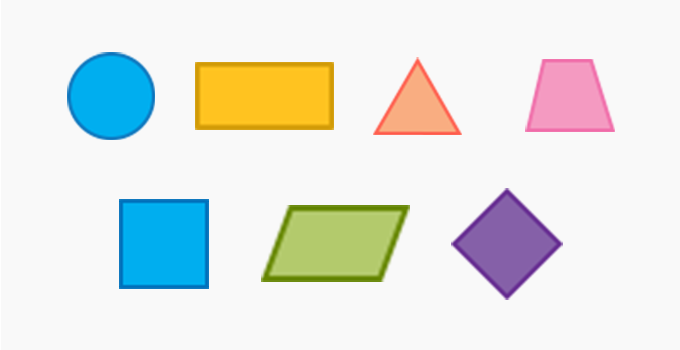Name & Shape Properties Area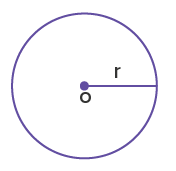Circle The length between the center “o” to the point on the circumference is the radius “r” A = πr2  π = 3.14 (constant)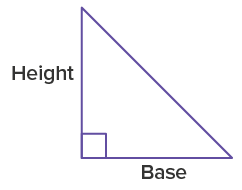Triangle One angle is the right angle A = 1⁄2 × Base × Height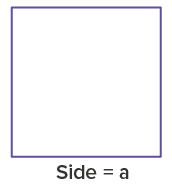Square All sides are equal Each angle is 90 degree A = Length of Side2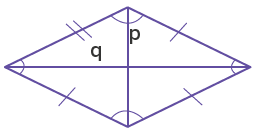Rhombus All sides are equal Opposite sides are parallel Opposite angles are equal A = p × qRectangle Opposite sides are equal and parallel Each angle is 90 degree A = Length × Breadth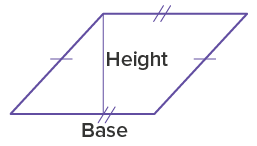Parallelogram Opposite sides are equal and parallel Opposite angles are equal A = Base × Height

The “textured” region represents the area of the shape

## Unit of measurement

The unit of measurement for the area is always the square of the unit in which lengths are given. The resultant unit is the product of the units of the given lengths.

Let’s take an example, the area of a square with side length 8 cm is:

Area = (Length of side)2

Area = 8 cm × 8 cm

Area = 64 cm2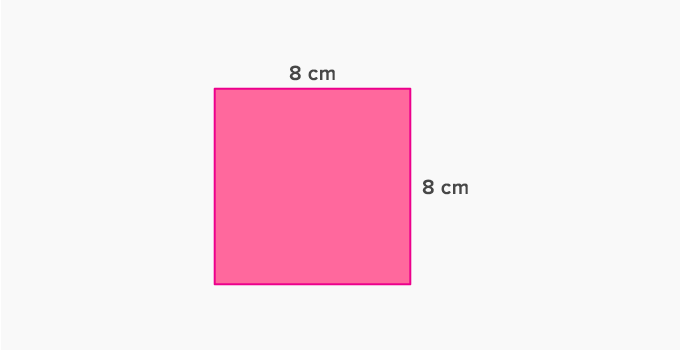Application

The application field of area formula is in architecture, land surveying, and map designing. The rescaled version of the area for a given place is useful in designing knowledge tools such as globes and geophysical maps. The area calculation for a two-dimensional shape is the first step to interpret the volume of a three-dimensional object such as cone, cylinder, ball, and cube.

 Fun Facts The Babylonian clay tablets with scribes were used to study math and interpret the concept of area using formula during 1800-1600 BCE Egyptians built great pyramids using the area based properties and ratio between the surface area of sides and base area for a perfectly balanced structure

Related math vocabulary

Won Numerous Awards & Honors